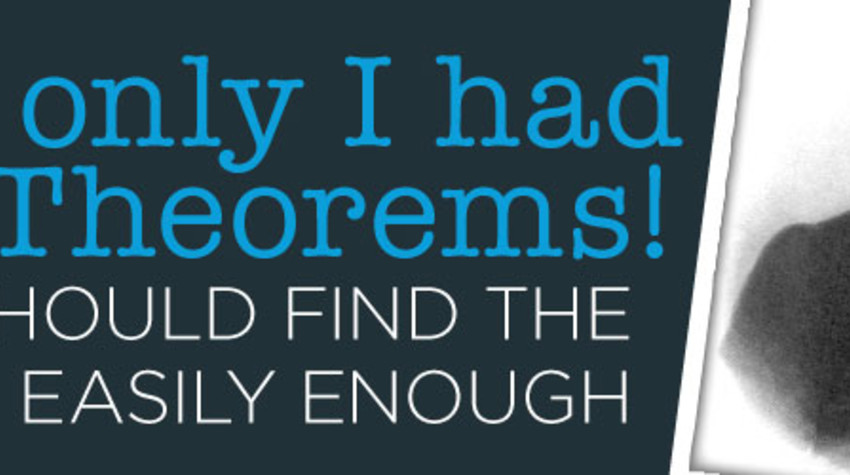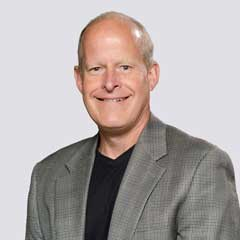# The Hidden Gems of Mathematics: Riemann (4 of 4)

April 16 2019 • Dave KesterThis is the final part of a 4-part series where I share how math has changed my career and my life. I’m sharing 3 heroes of mine in this series that have impacted actuarial science, mathematics, and many parts of our lives. I discussed my first two heroes, Leonhard Euler and Carl Gauss, in the previous two blogs.

Hero #3 is Bernhard Riemann, born in modern-day Germany September 17, 1826, and died July 20, 1866.

For starters, when I studied for the actuarial exams, we used Riemann sums to approximate integrals by using a finite sum. This technique is still valid, though it’s not on the current exam syllabus. But Riemann’s most interesting work is in math theory. In my previous blog, I mentioned how Gauss tutored Riemann and Riemann extended much of Gauss’s work. Riemann, like Gauss, was born to a poor family. But, like Gauss, Riemann didn’t let lack of money get in the way of making history.

Riemann extended Gauss’s work with non-Euclidean geometry. Riemann created a geometry called the Riemann surfaces that built a physical bridge between light, matter, and space time. The cool thing about these surfaces is they can have more than 3 dimensions. With the Riemann surfaces, Riemann officially expanded geometry beyond Euclid. But, more importantly, Albert Einstein used Riemann’s non-Euclidean geometry in his theories of relativity. In other words, Einstein couldn’t have done his magic unless Riemann, Gauss, Euler, and other mathematicians did their magic. It’s difficult to measure the impact of Einstein’s work, which is also a measure of Riemann’s work. For starters, the GPS on your phone gives an accurate measurement of your location within yards. Without the work of Einstein, who relied on Riemann’s work, our GPS would be so inaccurate it would not be useful.

As mentioned in my previous blog, Gauss identified a formula for calculating the probability of a number being a prime number. What was missing from this formula was an error term. In identifying this error term, Riemann made his biggest splash. This splash came in the form of a short but historic 1859 paper. Riemann returned to the zeta function where Euler made history by connecting prime numbers to natural numbers: . Euler and other mathematicians studied this zeta function for s values where s is a real number. Riemann expanded the zeta function by considering complex numbers for s. Complex numbers have a real number part and an imaginary number part. Not only did Riemann extend the input to complex numbers, but he used analytic continuation for when the function is not defined to give results for s<1. The real action and the real mystery occurs when the real part for s is strictly between 0 and 1. The question is what values for s cause the zeta function to equal 0. In Riemann’s 1959 paper, he hypothesized that all values for s for which the zeta function is zero all have a real part 0.5 except for the “trivial zeros.”

This conjecture may be the most famous math problem yet unsolved. You can earn a million dollars if you prove this hypothesis. Progress has been made but no proof exists. However, it has been proven that all nontrivial zeros occur where the real part of s is between 0 and 1. One amazing result of his hypothesis is that it connects prime numbers to the complex plane. Remember prime numbers are discrete but the complex plane is continuous. Riemann connected discrete prime numbers to the continuous complex plane! Nobody saw that coming.

Also, no 19th century mathematician saw the impact prime numbers would have on our lives today. Internet security relies on prime numbers which is connected to the Riemann hypothesis. In addition, many mathematical proofs are based on the Riemann hypothesis. Therefore, the Riemann hypothesis is the foundation for many layers of math wonder built since that remarkable 1859 paper. Many interesting books have been written on Riemann. One of my favorite is The Music of the Primes by Marcus du Sautoy, who explains how Riemann’s hypothesis is like a great symphony unfolding. This was the book I mentioned in my first blog that sparked my interest in math history.

I’m sure some of you were surprised when I declared mathematicians as heroes. But the impact these three men have on our lives today cannot be overstated. Of course, I could include hundreds more mathematicians because the cool thing about math is math builds on past work. No mathematician is an island. Unlike much of science and technology, math is not dated. Once something is proven true mathematically, it is always true. Most mathematicians are quiet and humble. Some have weak social skills. Their discoveries do not make headline news. But the math, science, technology, and music we enjoy today are a result of many mathematicians making contributions through the ages.

However, much of the work mathematicians do is uncovering and understanding the math that already exists in the world and universe that we live in. Many of you may not be able to invest time in reading math books for fun. But we can all slow down and observe the shapes and patterns that we see in our world and appreciate them in a new light. I’ll finish with an example.

A sine wave may seem simple but has amazing properties. If we pair the triangle with a unit circle and add a little math magic, we can create a sine wave. Have you ever tried drawing a perfect sine wave? It’s difficult. And it’s beautiful. The cool thing is we know sine waves are everywhere in our universe, yet they often don’t come from triangles. We know sine waves are the basis for sound, light, electricity, and other natural phenomena. Here’s an example that you may not know. The number of sunlight hours we receive each day follows a sine wave. Think about that. The way the earth and sun move and dance together creates light for us each day that we can predict with certainty using a sine wave. If we know the shape and pattern of a sine wave, we know the pattern for how much light we enjoy each day. That means seemingly trivial facts, such as the sin(30)=0.5 now has new meaning. I’ll leave that thought as something for you to chew on. Math is making music everywhere. Let’s enjoy the music together!

This is the final part of a 4-part series where I share how math has changed my career and my life. I’m sharing 3 heroes of mine in this series that have impacted actuarial science, mathematics, and many parts of our lives. I discussed my first two heroes, Leonhard Euler and Carl Gauss, in the previous two blogs.

## Related Posts